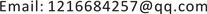1. 引言

2. 多震源混合信号概述

P b l = Γ P (1)

P b l j = ∑ k = 1 N Γ k j P k (2)

3. BSS简介3.1. BSS基本原理

x 1 ( t ) = a 11 s 1 ( t ) + a 12 s 2 ( t ) + a 13 s 3 ( t ) x 2 ( t ) = a 21 s 1 ( t ) + a 22 s 2 ( t ) + a 23 s 3 ( t ) x 3 ( t ) = a 31 s 1 ( t ) + a 32 s 2 ( t ) + a 33 s 3 ( t ) (3)

x i ( t ) − ∑ i = 1 N a i j s i ( t ) ,     i = 1 , 2 , ⋯ , N (4)

x i ( t ) − ∑ i = 1 N ∑ k = 0 P a i j s i ( t − k ) ,     i = 1 , 2 , ⋯ , N (5)

3.2. 信号预处理

3.2.1. 去均值过程

x ¯ i ( t ) = x i ( t ) − 1 N ∑ i = 1 N x i ( t ) ,     i = 1 , 2 , ⋯ , N (6)

3.2.2. 白化过程

E { x x T } = E D E T (7)

V = E D − 1 / 2 E T (8)

E { z z T } = A ˜ E { s s T } A ˜ T = I (9)

4. 算法介绍及数据模拟4.1. 线性混合盲分离算法

{ y 1 ( t ) = x 1 ( t ) − ω 1 x 2 ( t ) y 2 ( t ) = x 2 ( t ) − ω 2 x 1 ( t ) (10)

ω 1 = r x 1 y 2 ( t 1 , t ′ 1 ) r x 2 y 2 ( t 1 , t ′ 1 ) = r x 1 y 2 ( t 1 , τ 1 ) r x 2 y 2 ( t 1 , τ 1 ) (11)

ω 2 = r y 1 x 2 ( t 2 , t ′ 2 ) r y 1 x 1 ( t 2 , t ′ 2 ) = r y 1 x 2 ( t 2 , τ 2 ) r y 1 x 1 ( t 2 , τ 2 ) (12)

W = ( 1 − ω 1 − ω 2 1 ) (13)

W ( i j ) W → W

W ( i j ) = [ cos θ i j sin θ i j − sin θ i j cos θ i j ] (14)

4.2. 算法步骤

1) 将观测得到的混合数据用X表示；

2) 求出零时间延迟相关矩阵 R x x ( 0 ) = E [ X X T ] ；

3) 对 R x x ( 0 ) 进行奇异值分解，得到 R x x ( 0 ) = U A U ，U为正交矩阵，A为对角矩阵；

4) 计算预白化矩阵 B = A − 1 / 2 U T ，则得到预白化后和混合数据为 Z = B X ;

5) 初始化盲分离信号Y，选取一对成分 y i , y j ，计算角度 θ i j ；

6) 如果 θ i j > ε ，其中 ε ≪ 1 ，求出 W ( i j ) = [ cos θ i j sin θ i j − sin θ i j cos θ i j ] ，更新分离矩阵，

7) 旋转信号矩阵 Z : Z ← W ( i j ) Z ，直到 θ i j ≤ ε ，否则跳回(5)。

4.3. 数值模拟

5. 结语